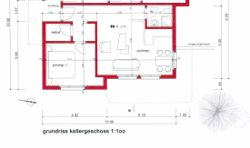deutsch | english | français

Bed and Breakfast Wallis » Visp » 2-Room-Apartment

# Apartment in Visp / Oberwallis / Wallis / SwitzerlandThe modern and new flat (2 rooms) with a patio is situated in Visp. The modern kitchen includes a dishwasher. TV (132 channels) and access to internet via WLAN come as part of the package as well as a clothes washer.

• Free parking: Yes
• Walking distance to central station: about 10 minutes
• 4 persons max

## Prices

• One night is 94 Swiss Francs / from 30 nights: 74 Swiss Francs / from 90 nights: 54 Swiss Francs
• Cleaning fee: 100 Swiss Francs
• Tourism fee: 2 Swiss Francs per person and day (children: 1 Swiss Franc)
• At least 7 days

## Availability

 2022 1 2 3 4 5 6 7 8 9 10 11 12 13 14 15 16 17 18 19 20 21 22 23 24 25 26 27 28 29 30 31 June x x July x x x x x x x x x x x x x x x x x x x x x x x x x x x x x x x August x x x x x x x x x x x x x x x x x x x x x x x x x x x x x x x September x x x x x x x x x x x x x x x x x x x x x x x x x x x x x x October x x x x x x x x x x x x x x x x x x x x x x x x x x x x x x x November x x x x x x x x x x x x x x x x x x x x x x x x x x x x x x December x x x x x x x x x x x x x x x x x x x x x x x x x x x x x x x